# Model Predictive Control of a Multi-Input Single-Output Plant

This example shows how to design a model predictive controller for a plant with one measured output, one manipulated variable, one measured disturbance, and one unmeasured disturbance.

### Define Plant Model

Define a plant model. For this example use continuous time transfer functions from each input to the output. Display the plant model at the command line.

```plantTF = tf({1,1,1},{[1 .5 1],[1 1],[.7 .5 1]}) % display transfer functions ```
```plantTF = From input 1 to output: 1 --------------- s^2 + 0.5 s + 1 From input 2 to output: 1 ----- s + 1 From input 3 to output: 1 ------------------- 0.7 s^2 + 0.5 s + 1 Continuous-time transfer function. ```

For this example, explicitly convert the plant to a discrete-time state space form before passing in to the MPC controller creation function.

The controller creation function can accept either continuous-time or discrete-time plants. When the optimization problem to find the optimal value of the manipulated variable is set up, the MPC controller automatically converts a continuous-time plant (in any format) to a discrete-time state space model, using Zero Order Hold.

Converting the plant to discrete-time is useful when you need the discrete-time system matrices for analysis or simulation (as in this example) or want the controller to use a discrete-time plant converted with a method other than ZOH.

```plantCSS = ss(plantTF); % convert plant from transfer function to continuous-time state space Ts = 0.2; % specify a sample time of 0.2 seconds plantDSS = c2d(plantCSS,Ts); % convert plant to discrete-time state space, using Zero Order Hold ```

By default, all the plant input signals are assumed to be manipulated variables. Use `setmpcsignal` to specify which signals are measured and unmeasured disturbances. In this example, the first input signal is a manipulated variable, the second is a measured disturbance, the third one is an unmeasured disturbance. This information is stored in the plant model `plantDSS` and later used by the mpc controller.

```plantDSS = setmpcsignals(plantDSS,'MV',1,'MD',2,'UD',3); % specify signal types ```

### Design MPC Controller

Create the controller object, specifying the sampling time, as well as the prediction and control horizons (10 and 3 steps respectively).

```mpcobj = mpc(plantDSS,Ts,10,3); ```
```-->The "Weights.ManipulatedVariables" property of "mpc" object is empty. Assuming default 0.00000. -->The "Weights.ManipulatedVariablesRate" property of "mpc" object is empty. Assuming default 0.10000. -->The "Weights.OutputVariables" property of "mpc" object is empty. Assuming default 1.00000. ```

Because you have not specified the weights of the quadratic cost function to be minimized by the controller, their value is assumed to be the default one (0 for the manipulated variables, 0.1 for the manipulated variable rates, 1 for the output variables). Also, at this point the MPC problem is still unconstrained as you have not specified any constraint yet.

Define hard constraints on the manipulated variable.

```mpcobj.MV = struct('Min',0,'Max',1,'RateMin',-10,'RateMax',10); ```

The input and output disturbance models specify the dynamic characteristics of the unmeasured disturbances on the input and output, respectively, so they can be better rejected. By default, these disturbance models are assumed to be integrators unless you specify them otherwise. The mpc object also has a noise model that specifies the dynamics of the noise on the measured output variable. By default this is assumed to be a unity static gain, which is equivalent to assume that the noise is white. In this example, there is no measured output disturbance, so there is no need to specify an output disturbance model, and the noise on the measured output signal is assumed to be white.

Specify the disturbance model for the unmeasured input as an integrator driven by white noise with variance = `1000`.

```mpcobj.Model.Disturbance = tf(sqrt(1000),[1 0]); ```

Display the MPC controller object `mpcobj` to review its properties.

```mpcobj ```
``` MPC object (created on 23-Feb-2021 13:06:50): --------------------------------------------- Sampling time: 0.2 (seconds) Prediction Horizon: 10 Control Horizon: 3 Plant Model: -------------- 1 manipulated variable(s) -->| 5 states | | |--> 1 measured output(s) 1 measured disturbance(s) -->| 3 inputs | | |--> 0 unmeasured output(s) 1 unmeasured disturbance(s) -->| 1 outputs | -------------- Indices: (input vector) Manipulated variables: [1 ] Measured disturbances: [2 ] Unmeasured disturbances: [3 ] (output vector) Measured outputs: [1 ] Disturbance and Noise Models: Output disturbance model: default (type "getoutdist(mpcobj)" for details) Input disturbance model: user specified (type "getindist(mpcobj)" for more details) Measurement noise model: default (unity gain after scaling) Weights: ManipulatedVariables: 0 ManipulatedVariablesRate: 0.1000 OutputVariables: 1 ECR: 100000 State Estimation: Default Kalman Filter (type "getEstimator(mpcobj)" for details) Constraints: 0 <= MV1 <= 1, -10 <= MV1/rate <= 10, MO1 is unconstrained ```

Display the input disturbance model. As expected is the specified integrator converted to discrete time.

```getindist(mpcobj) ```
```ans = A = x1 x1 1 B = Noise#1 x1 0.8 C = x1 UD1 7.906 D = Noise#1 UD1 0 Sample time: 0.2 seconds Discrete-time state-space model. ```

Display the output disturbance model. As expected it is empty.

```getoutdist(mpcobj) ```
``` Assuming no disturbance added to measured output channel #1. -->The "Model.Noise" property of the "mpc" object is empty. Assuming white noise on each measured output channel. ans = Empty state-space model. ```

To examine whether the MPC controller will be able to reject constant output disturbances and track constant setpoint with zero offsets in steady-state, compute the closed loop DC gain from output disturbances to controlled outputs using the `cloffset` command. This is also known as the steady state output sensitivity of the closed loop.

```DC = cloffset(mpcobj); fprintf('DC gain from output disturbance to output = %5.8f (=%g) \n',DC,DC); ```
```DC gain from output disturbance to output = 0.00000000 (=5.77316e-15) ```

A zero gain (which is typically the result of the controller having integrators as input or output disturbance models) means that the measured plant output will track the desired output reference setpoint.

### Simulate Closed-Loop Response Using the `sim` Command

The `sim` command provides a quick way to simulate an MPC controller in a closed loop with a linear time-invariant plant when constraints and weights stay constants and the disturbance and reference signals can be easily and completely specified ahead of time.

First, specify simulation time and the reference and disturbance signals

```Tstop = 30; % simulation time Nf = round(Tstop/Ts); % number of simulation steps r = ones(Nf,1); % output reference signal v = [zeros(Nf/3,1);ones(2*Nf/3,1)]; % measured input disturbance signal ```

Run the closed-loop simulation and plot results. The plant specified in `mpcobj.Model.Plant` is used both as a plant model in the closed loop and as the internal plant model used by the controller to predict the response over the prediction horizon. Use `sim` to simulate the closed loop system for Nf steps with reference r and measured input disturbance v.

```sim(mpcobj,Nf,r,v) % simulate plant and controller in closed loop ```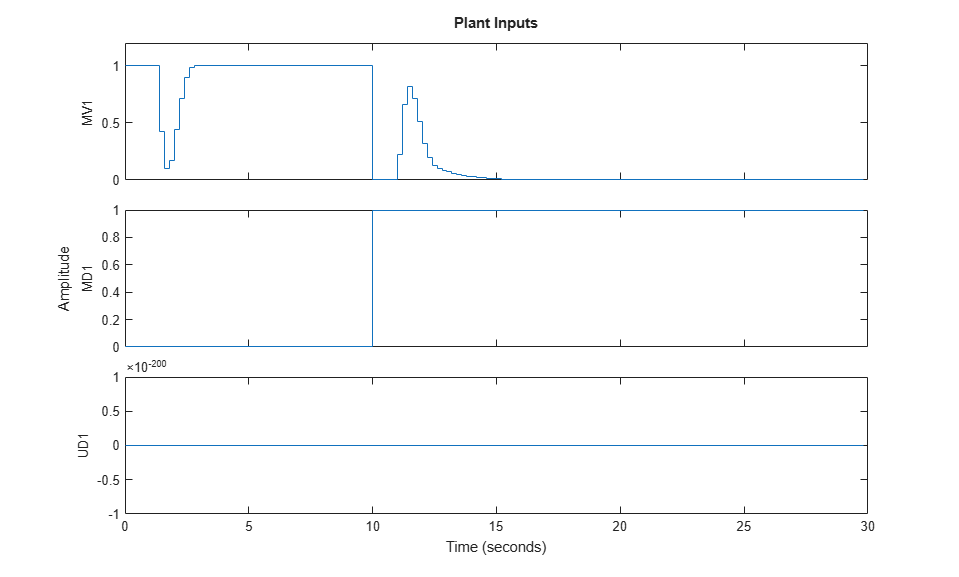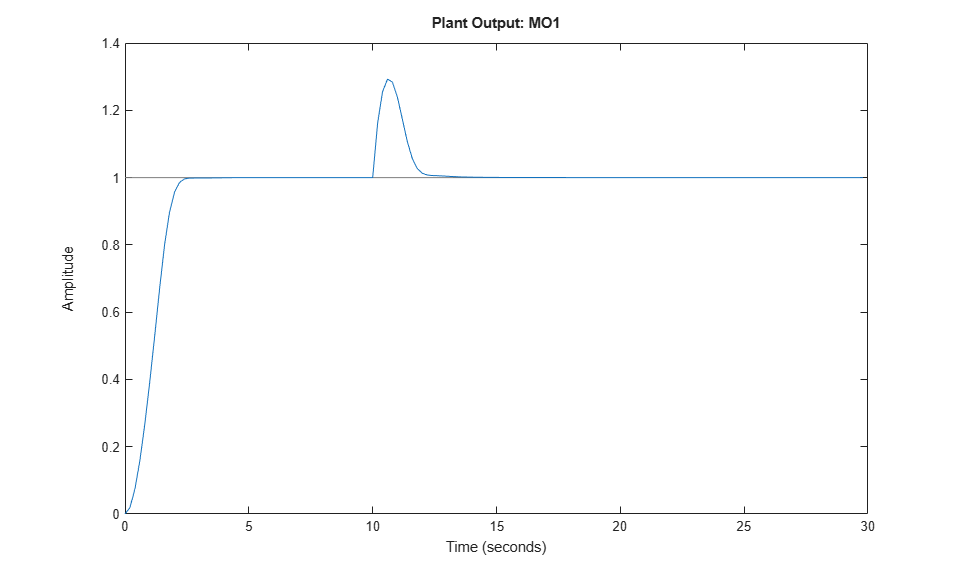The manipulated variable hits the upper bound initially, and brings the plant output to the reference value within a few seconds. It then settles in at its maximum allowed value, 1. After 10 seconds, the measured disturbance signal rises from 0 to 1, which causes the plant output to exceed its reference value by about 30%. The manipulated variable hits the lower bound in an effort to reject the disturbance. The controller is able to bring the plant output back to the reference value after a few seconds, and the manipulated variable settles in at its minimum value. The unmeasured disturbance signal is always zero, because no unmeasured disturbance has been specified yet.

You can use a simulation options objects to specify additional simulation options as well as noise and disturbance signals that feed into the plant but are unknown to the controller. For this example, use a simulation option object to specify the unmeasured input disturbance signal as well as additive noise on both manipulated variables and measured outputs. You will also later use this options object to specify a plant to be used in simulation, which differs from the one used internally by the controller. Create a simulation option object with default options and no signal.

```SimOptions = mpcsimopt; % create object ```

Create a disturbance signal and specify it in the simulation options object

```d = [zeros(2*Nf/3,1);-0.5*ones(Nf/3,1)]; % define a step disturbance signal SimOptions.UnmeasuredDisturbance = d; % specify unmeasured input disturbance signal ```

Specify noise signals in the simulation options object. At simulation time, the simulation function directly adds the specified output noise to the measured output before feeding it to the controller. It also directly adds the specified input noise to the manipulated variable (not to any disturbance signals) before feeding it to the plant.

```SimOptions.OutputNoise=.001*(rand(Nf,1)-.5); % specify output measurement noise SimOptions.InputNoise=.05*(rand(Nf,1)-.5); % specify noise on manipulated variables ```

Note that you can also use the `OutputNoise` field of the simulation option object to specify a more general additive output disturbance signal (such as a step, for example) on the measured plant output.

Use `sim` with the additional `SimOptions` argument to simulate the closed loop system and save the results to the workspace variables `y`,|t|,|u|, and `xp`. This allows you to selectively plot signals in a new figure window and in any given color and order.

```[y,t,u,xp] = sim(mpcobj,Nf,r,v,SimOptions); % simulate closed loop ```

Plot results.

```figure % create new figure subplot(2,1,1) % create upper subplot plot(0:Nf-1,y,0:Nf-1,r) % plot plant output and reference title('Output') % add title so upper subplot ylabel('MO1') % add a label to the upper y axis grid % add a grid to upper subplot subplot(2,1,2) % create lower subplot plot(0:Nf-1,u) % plot manipulated variable title('Input'); % add title so lower subplot xlabel('Simulation steps') % add a label to the lower x axis ylabel('MV1') % add a label to the lower y axis grid % add a grid to lower subplot ```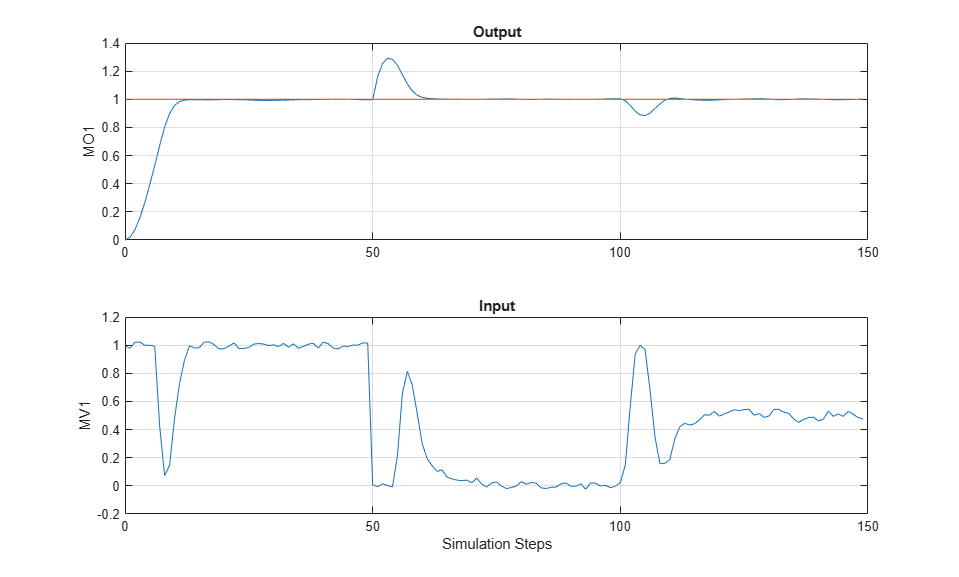Despite the added noise (which is especially visible on the manipulated variable plot) and despite the measured and unmeasured disturbances kicking in after 50 and 100 steps respectively, the controller is able to achieve and maintain good tracking. The manipulated variable settles in at about 1 after the initial part of the simulation (steps from 20 to 50), at about 0 to reject the measured disturbance (steps from 70 to 100), and at about 0.5 to reject both disturbances (steps from 120 to 150).

### Simulate Closed-Loop Response with Model Mismatch

Test the robustness of the MPC controller against a model mismatch. Specify the true plant to be used in simulation as `truePlantCSS`.

```truePlantTF = tf({1,1,1},{[1 .8 1],[1 2],[.6 .6 1]}) % specify and display transfer functions truePlantCSS = ss(truePlantTF); % convert to continuous state space truePlantCSS = setmpcsignals(truePlantCSS,'MV',1,'MD',2,'UD',3); % specify signal types ```
```truePlantTF = From input 1 to output: 1 --------------- s^2 + 0.8 s + 1 From input 2 to output: 1 ----- s + 2 From input 3 to output: 1 ------------------- 0.6 s^2 + 0.6 s + 1 Continuous-time transfer function. ```

Update the simulation option object by specifying `SimOptions.Model` as a structure with two fields, `Plant` (containing the true plant model) and `Nominal` (containing the operating point values for the true plant). For this example, the nominal values for the state derivatives and the inputs are not specified, so they are assumed to be zero, resulting in `y = SimOptions.Model.Nominal.Y + C*(x-SimOptions.Model.Nominal.X)` where `x` and `y` are the state and measured output of the plant, respectively.

```SimOptions.Model = struct('Plant',truePlantCSS); % create the structure and assign the 'Plant' field SimOptions.Model.Nominal.Y = 0.1; % create and assign the 'Nominal.Y' field SimOptions.Model.Nominal.X = -.1*[1 1 1 1 1]; % create and assign the 'Nominal.X' field SimOptions.PlantInitialState = [0.1 0 -0.1 0 .05]; % specify the initial state of the true plant ```

remove any signal previously added to the measured output and to the manipulated variable

```SimOptions.OutputNoise = []; % remove output measurement noise SimOptions.InputNoise = []; % remove noise on manipulated variable ```

Run the closed-loop simulation and plot the results. Since `SimOptions.Model` is not empty, `SimOptions.Model.Plant` is converted to discrete time (using zero order hold) and used as the plant model in the closed loop simulation, while the plant in `mpcobj.Model.Plant` is only used by the controller to predict the response over the prediction horizon.

```sim(mpcobj,Nf,r,v,SimOptions) % simulate the closed loop ```
```-->Converting model to discrete time. ```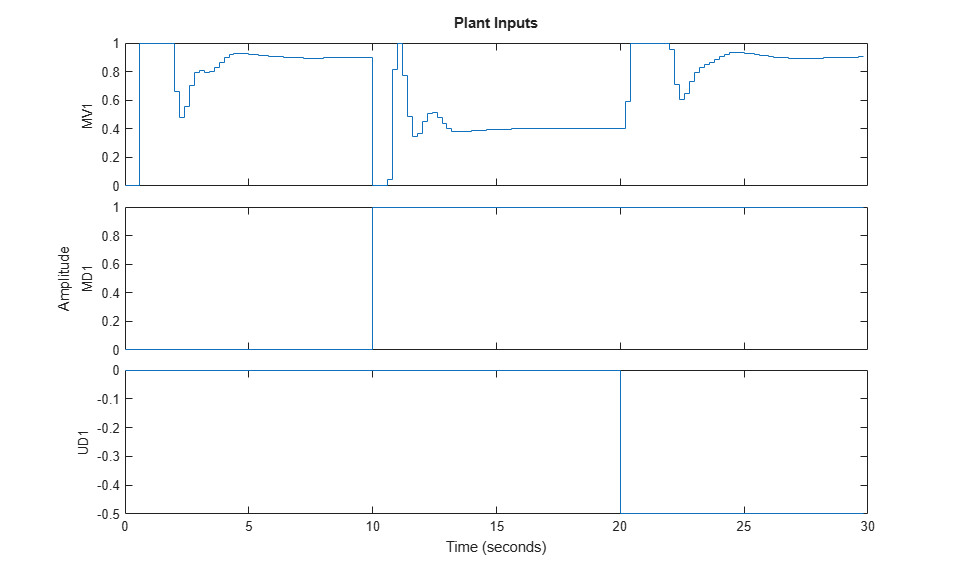As a result of the model mismatch, some degradation in the response is visible, notably the controller needs a little more time to achieve tracking and the manipulated variable now settles at about 0.5 to reject the measured disturbance (see values from 5 to 10 seconds) and settles at about 0.9 to reject both input disturbances (from 25 to 30 seconds). Despite this, the controller is still able to track the output reference.

### Soften Constraints

Every constraint is associated to a dimensionless parameter called ECR value. A constraint with larger ECR value is allowed to be violated more than a constraint with smaller ECR value. By default all constraints on the manipulated variables have an ECR value of zero, making them hard. You can specify a nonzero ECR value for a constraint to make it soft.

Note that is possible to refer to the `ManipulatedVariables` field also as `MV`. Relax the constraints on manipulated variables from hard to soft.

```mpcobj.MV.MinECR = 1; % ECR for the lower bound on the manipulated variable mpcobj.MV.MaxECR = 1; % ECR for the upped bound on the manipulated variable ```

Define an output constraint. By default all constraints on output variables (measured outputs) have an ECR value of one, making them soft. You can reduce the ECR value for an output constraint to make it harder, however it is always advised to keep output constraints soft. This is mainly because the plant outputs depend on plant states as well as on the measured disturbances, therefore, if a large disturbance occurs, the plant outputs can violate the constraints independently on any control action taken by the mpc controller, especially when the manipulated variables are themselves hard bounded. Such an unavoidable violation of an hard constraint results in an infeasible MPC problem, for which no MV can be calculated.

```mpcobj.OV.Max = 1.1; % define the (soft) output constraint % Note that is possible to refer to the |OutputVariables| field also as |OV|. ```

Run a new closed-loop simulation, without including the simulation option object, and therefore without any model mismatch, unmeasured disturbance, or noise added to the manipulated variable or measured output.

```sim(mpcobj,Nf,r,v) % simulate the closed loop ```
``` Assuming no disturbance added to measured output channel #1. -->The "Model.Noise" property of the "mpc" object is empty. Assuming white noise on each measured output channel. ```In an effort to reject the measured disturbance, achieve tracking, and prevent the output to rises above its 1.1 soft constraint, the controller slightly violates the soft constraint on the manipulated variable, which reaches small negative values from seconds 10 to 11 (you can zoom in the picture to see this violation more clearly). The constraint on the measured output is violated more than the one on the manipulated variable.

Penalize more the output constraint violations and rerun the simulation.

```mpcobj.OV.MaxECR = 0.001; % the closer to zero, the harder the constraint sim(mpcobj,Nf,r,v) % run a new closed-loop simulation. ```
``` Assuming no disturbance added to measured output channel #1. -->The "Model.Noise" property of the "mpc" object is empty. Assuming white noise on each measured output channel. ```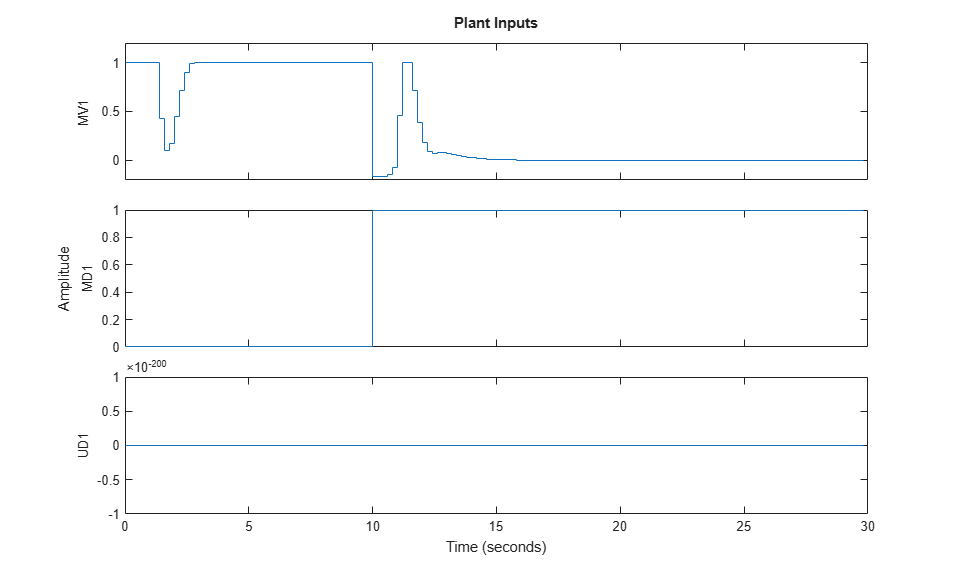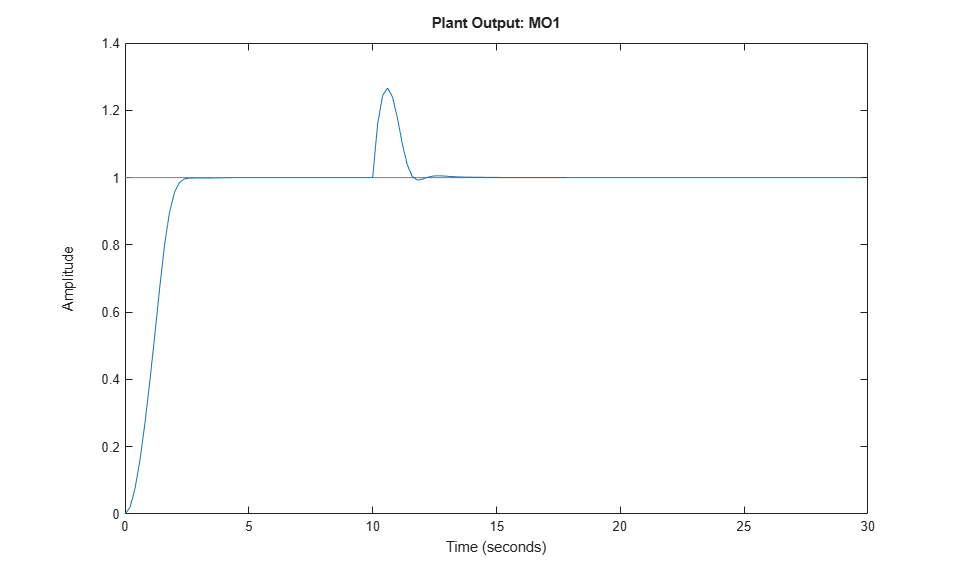Now the controller violates the output constraint only very slighly. As expected, this output performance improvement comes at the cost of violating the manipulated variable constraint a lot more (the manipulated variable reaches -3 for a couple of steps).

### Change Kalman Gains Used in the Built-In State Estimator

At each time step, the MPC controller obtains the manipulated variable by multiplying the discrete-time plant state (if available) by a gain matrix (computed by solving a constrained quadratic optimization problem). Since the plant state is normally not available, by default, the controller uses a linear Kalman filter as an observer to estimate the state of the discrete-time augmented plant (that is the plant comprehensive of the disturbance and noise models). Therefore, the states of the controller are the states of this Kalman filter, which are in turn the estimates of the states of the augmented discrete-time plant.

Run a closed loop simulation with model mismatch and unmeasured disturbance, using the default estimator, and return the controller states structure `xc` in the workspace, so you can later compare the state sequences.

```[y,t,u,xp,xc] = sim(mpcobj,Nf,r,v,SimOptions); ```
```-->Converting model to discrete time. ```

display the component of the controller states structure

```xc ```
```xc = struct with fields: Plant: [150x5 double] Disturbance: [150x1 double] Noise: [150x0 double] LastMove: [150x1 double] ```

Plot the plant output response as well as the plant states estimated by the default observer

```figure; % create figure subplot(2,1,1) % create upper subplot axis plot(t,y) % plot y versus time title('Plant output'); % add title to upper plot ylabel('y') % add a label to the upper y axis grid % add grid to upper plot subplot(2,1,2) % create lower subplot axis plot(t,xc.Plant) % plot xc.Plant versus time title('Estimated plant states'); % add title to lower plot xlabel('Time (sec)') % add a label to the lower x axis ylabel('xc') % add a label to the lower y axis grid % add grid to lower plot ```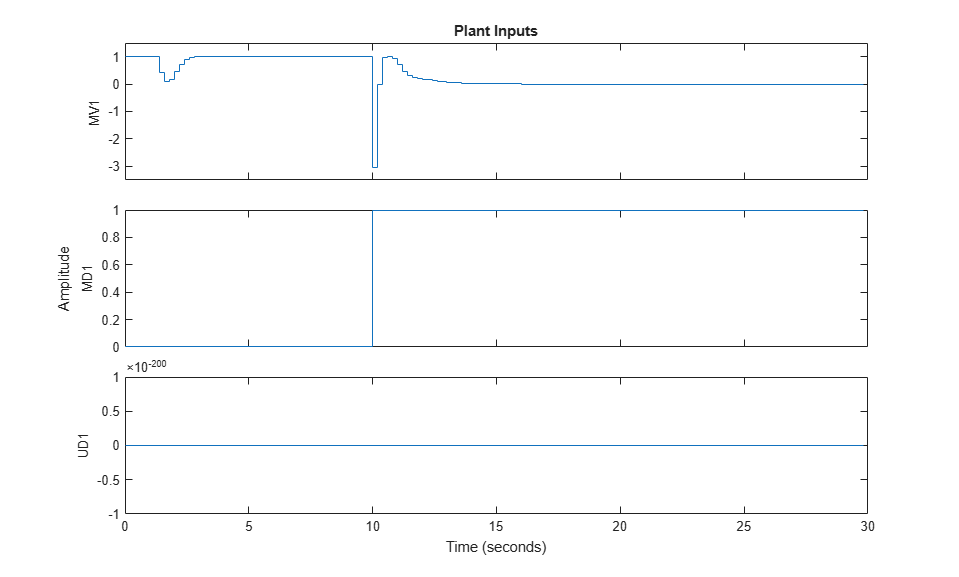As expected, there are sudden changes at 10 and 20 seconds due to the measured and unmeasured disturbance signals kicking in, respectively.

You can change the gains of the Kalman filter used as observer. To do so, first, retrieve the default Kalman gains and state-space matrices.

```[L,M,A1,Cm1] = getEstimator(mpcobj); % retrieve observer matrices ```

Calculate and display the poles of the default observer. Note that, as expected, they are all inside the unit circle, though a few of them seem relatively close to the border. Note that there are six states, five belonging to the plant model, the sixth belonging to the input disturbance model.

```e = eig(A1-A1*M*Cm1) % eigenvalues of observer state matrix ```
```e = 0.5708 + 0.4144i 0.5708 - 0.4144i 0.4967 + 0.0000i 0.9334 + 0.1831i 0.9334 - 0.1831i 0.8189 + 0.0000i ```

Design a new state estimator by pole-placement.

```poles = [.8 .75 .7 .85 .6 .81]; % specify desired positions for the new poles L = place(A1',Cm1',poles)'; % calculate Kalman gain for time update M = A1\L; % calculate Kalman gain for measurement update ```

Set the new matrix gains in the mpc controller object

```setEstimator(mpcobj,L,M); % set the new estimation gains ```

re-run the closed loop simulation

```[y,t,u,xp,xc] = sim(mpcobj,Nf,r,v,SimOptions); ```
``` Assuming no disturbance added to measured output channel #1. -->The "Model.Noise" property of the "mpc" object is empty. Assuming white noise on each measured output channel. -->Converting model to discrete time. ```

Plot the plant output response as well as the plant states estimated by the new observer

```figure; % create figure subplot(2,1,1) % create upper subplot axis plot(t,y) % plot y versus time title('Plant output'); % add title to upper plot ylabel('y') % add a label to the upper y axis grid % add grid to upper plot subplot(2,1,2) % create lower subplot axis plot(t,xc.Plant) % plot xc.Plant versus time title('Estimated plant states'); % add title to lower plot xlabel('Time (sec)') % add a label to the lower x axis ylabel('xc') % add a label to the lower y axis grid % add grid to lower plot ```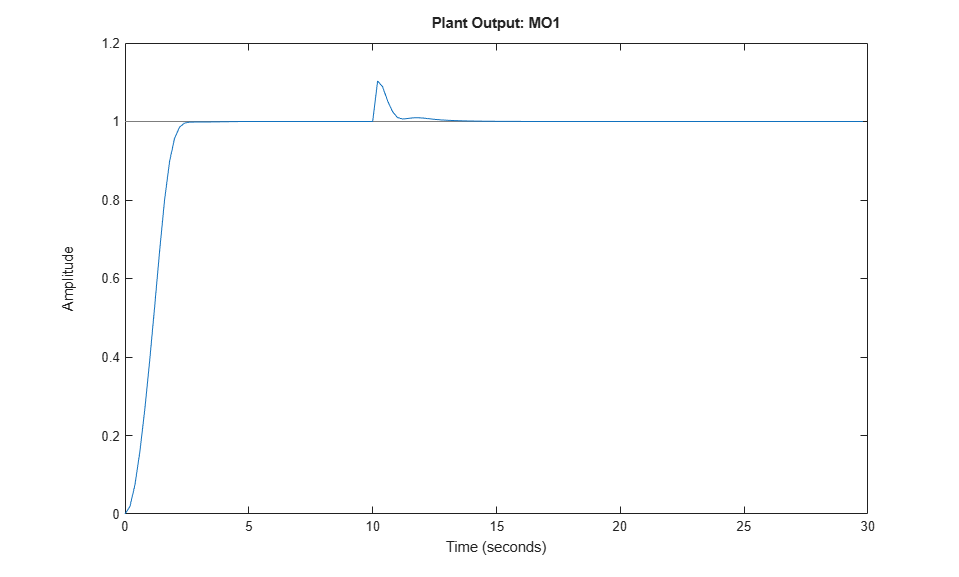As expected the controller states are different from the ones previously plotted, and the overall closed loop response is somewhat slower.

### Simulate Open-Loop Response

Test the behavior of the plant and controller in open-loop, using the `sim` command. Set the `OpenLoop` flag to `on`, and provide the sequence of manipulated variables to excite the system.

```SimOptions.OpenLoop = 'on'; % set open loop option SimOptions.MVSignal = sin((0:Nf-1)'/10); % define the manipulated variable signal ```

Simulate the open loop system containing the true plant (previously specified in `SimOptions.Model`) followed by the controller. As the reference signal will be ignored, you can avoid specifying it.

```sim(mpcobj,Nf,[],v,SimOptions) % simulate the open loop system ```
```-->Converting model to discrete time. ```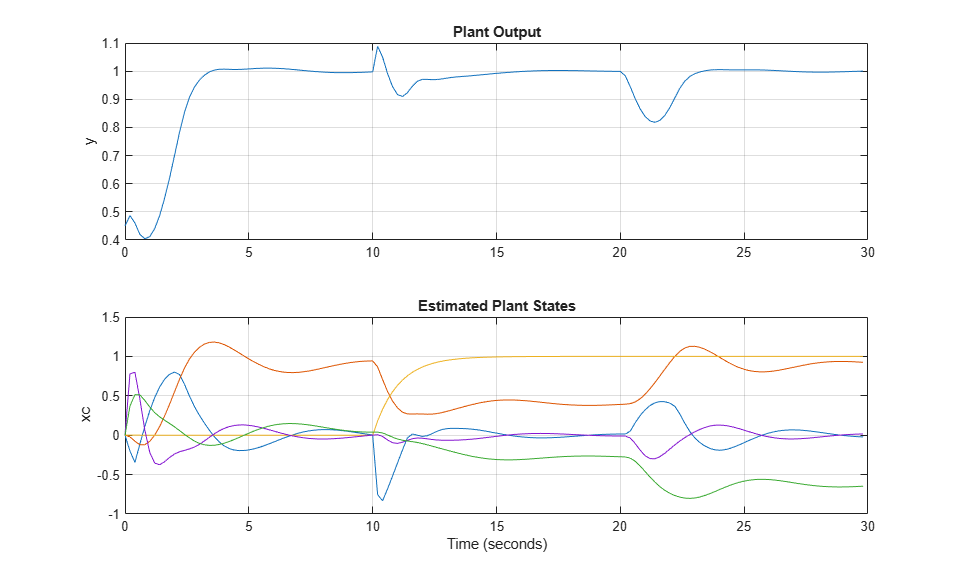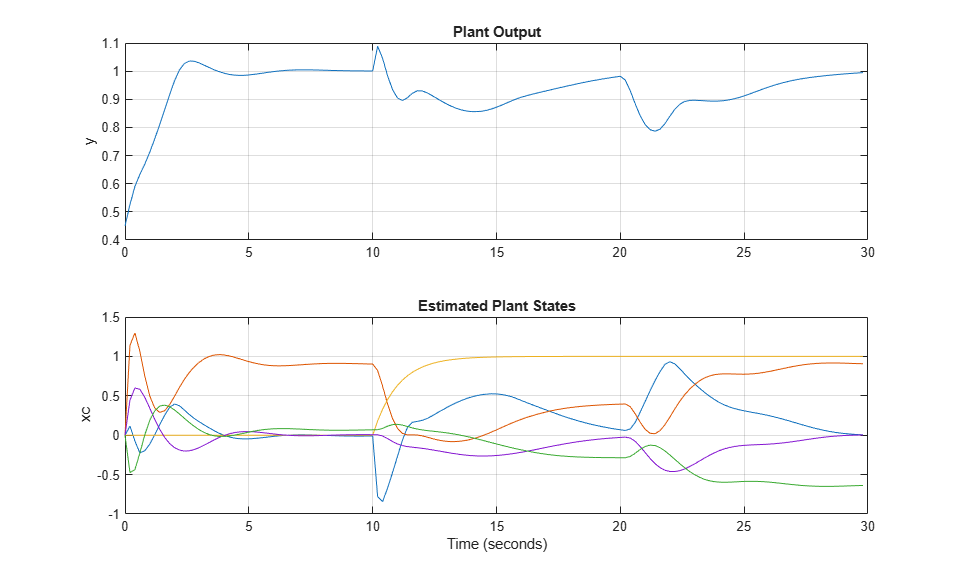### Simulate Controller in Closed Loop Using `mpcmove`

In the more general case of a closed loop in which the controller is applied to a nonlinear or time varying plant, constraints or weights can change at run time, or the disturbance and reference signals are hard to specify completely ahead of time, you can use the `mpcmove` function at each step in a for loop to simulate the MPC controller in closed loop. If your plant is continuous-time, you will need to convert it to discrete-time (using any method) to simulate it with a for loop.

First, obtain the discrete-time state-space matrices of the plant, and define simulation time and initial states for plant and controller.

```[A,B,C,D] = ssdata(plantDSS); % discrete-time plant plant ss matrices Tstop = 30; % Simulation time x = [0 0 0 0 0]'; % Initial state of the plant xmpc = mpcstate(mpcobj); % Initial state of the MPC controller r = 1; % Output reference signal ```

Display the initial state of the controller. Note that this is an `mpcstate` object (not a simple structure) and contains the controller states only at the current time (not the whole history up to the current time, as the structure returned by sim). It also contains the estimator covariance matrix. At each simulation step, you need to update `xmpc` with the new controller states.

```xmpc % controller states ```
```MPCSTATE object with fields Plant: [0 0 0 0 0] Disturbance: 0 Noise: [1x0 double] LastMove: 0 Covariance: [] ```

Define workspace arrays `YY` and `UU` to store the closed-loop trajectories so that they can be plotted after the simulation.

```YY=[]; UU=[]; ```

Run the simulation loop

```for k=0:round(Tstop/Ts)-1 % Define measured disturbance signal v(k). You might specify a more % complex dependence on time or previous states here. v = 0; if k*Ts>=10 % raising to 1 after 10 seconds v = 1; end % Define unmeasured disturbance signal d(k). You might specify a more % complex dependence on time or previous states here. d = 0; if k*Ts>=20 % falling to -0.5 after 20 seconds d = -0.5; end % Plant equations: current output % If you have a more complex plant output behavior (including, for example, % model mismatch or nonlinearities) you might want to simulate it here. % Note that there cannot be any direct feedthrough between u and y. y = C*x + D(:,2)*v + D(:,3)*d; % calculate current output (D(:,1)=0) YY = [YY,y]; % store current output % Compute the MPC action (u) and update the controller states (xmpc) using mpcmove % Note that xmpc is updated by mpcmove even though it is an input argument! u = mpcmove(mpcobj,xmpc,y,r,v); % xmpc,y,r,v are values at current step k % Plant equations: state update % You might want to simulate a more complex plant state behavior here. x = A*x + B(:,1)*u + B(:,2)*v + B(:,3)*d; % calculate next state and update current state UU = [UU,u]; % store current input end ```

Plot the results.

```figure % create figure subplot(2,1,1) % create upper subplot axis plot(0:Ts:Tstop-Ts,YY) % plot YY versus time ylabel('y') % add a label to the upper y axis grid % add grid to upper plot title('Output') % add title to upper plot subplot(2,1,2) % create lower subplot axis plot(0:Ts:Tstop-Ts,UU) % plot UU versus time ylabel('y') % add a label to the lower y axis xlabel('Time (sec)') % add a label to the lower x axis grid % add grid to lower plot title('Input') % add title to lower plot ```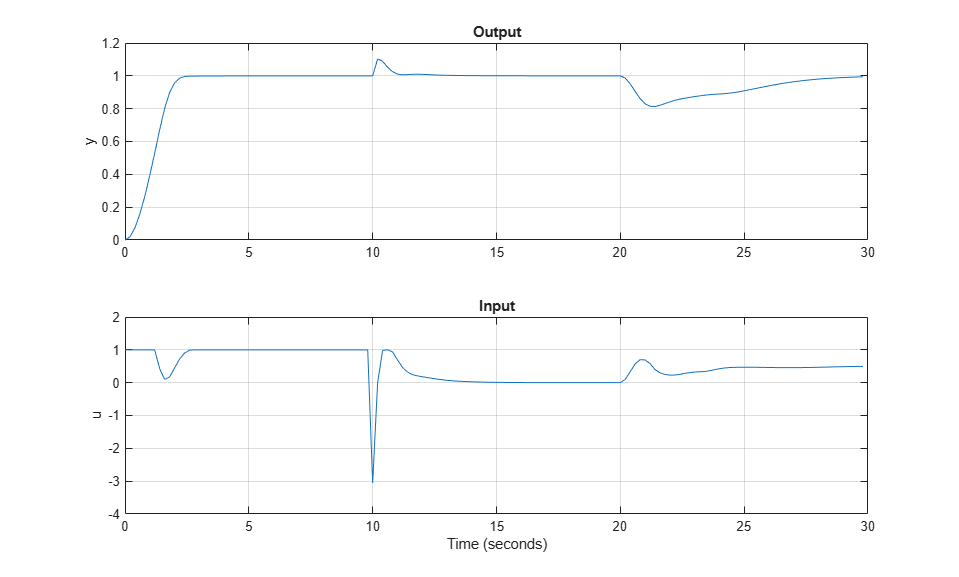If at any time during the simulation you want to check the optimal predicted trajectories from that point, they can be returned by `mpcmove` as well. Assume you start from the current state (`x` and `xmpc`), while the reference set-point changes to 0.5 and that the disturbance is gone (and that both signals remain constant during the prediction horizon).

```r = 0.5; % reference v = 0; % disturbance [~,info] = mpcmove(mpcobj,xmpc,y,r,v); % solve over prediction horizon ```

Display the info variable.

```info ```
```info = struct with fields: Uopt: [11x1 double] Yopt: [11x1 double] Xopt: [11x6 double] Topt: [11x1 double] Slack: 0 Iterations: 1 QPCode: 'feasible' Cost: 0.1399 ```

`info` is a structure containing the predicted optimal sequences of manipulated variables, plant states, and outputs over the prediction horizon. This sequence is calculated, together with the optimal move, by solving a quadratic optimization problem to minimize the cost function. The plant states and outputs in `info` result from applying the optimal manipulated variable sequence directly to `mpcobj.Model.Plant`, in an open-loop fashion. Therefore, this open-loop optimization process is therefore not equivalent to simulating the closed loop consisting of plant, estimator and controller using either the `sim` command or `mpcmove` iteratively in a for loop.

Extract the predicted optimal trajectories.

```topt = info.Topt; % time yopt = info.Yopt; % predicted optimal plant model outputs uopt = info.Uopt; % predicted optimal mv sequence ```

Plot the trajectories using stairstep plots. The stairstep plots are used because a normal plot would simply join each calculated point with the following one using a direct straight line, while the optimal discrete-time signals jump instantly at each step and stay constant until the next step. This difference is especially visible since there are only 10 intervals to be plotted for the plant output (and only 3 for the manipulated variable).

```figure % create new figure subplot(2,1,1) % create upper subplot stairs(topt,yopt) % plot yopt in a stairstep graph title('Optimal sequence of predicted outputs') % add title to upper subplot grid % add grid to upper subplot subplot(2,1,2) % create lower subplot stairs(topt,uopt) % plot uopt in a stairstep graph title('Optimal sequence of manipulated variables') % add title to upper subplot grid % add grid to upper subplot ```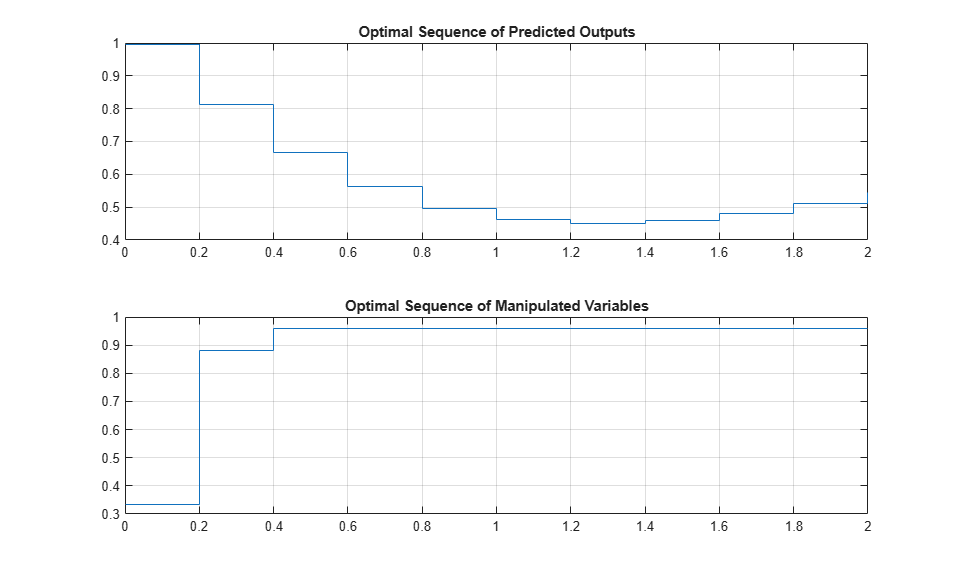### Linear Representation of MPC Controller

When the constraints are not active, the MPC controller behaves like a linear controller. Note that for a finite-time unconstrained Linear Quadratic Regulator problem with a finite non-receding horizon, the value function is time-dependent, which causes the optimal feedback gain to be time varying. In contrast, in MPC the horizon has a constant lenght, because it is always receding, resulting in a time-invariant value function, and consequently in a time-invariant optimal feedback gain.

You can get the state-space form of the MPC controller.

```LTI = ss(mpcobj,'rv'); % get state space representation ```

Get the state-space matrices to simulate the linearized controller.

```[AL,BL,CL,DL] = ssdata(LTI); % get state space matrices ```

Initialize variables for a closed loop simulation of both the original MPC controller without constraints and the linearized controller

```mpcobj.MV = []; % remove input constraints mpcobj.OV = []; % remove output constraints Tstop = 5; % set simulation time y = 0; % set nitial measured output r = 1; % set output reference set-point (constant) u = 0; % set previous (initial) input command x = [0 0 0 0 0]'; % set initial state of plant xmpc = mpcstate(mpcobj); % set initial state of unconstrained MPC controller xL = zeros(size(BL,1),1); % set initial state of linearized MPC controller YY = []; % define workspace array to store plant outputs ```
``` Assuming no disturbance added to measured output channel #1. -->The "Model.Noise" property of the "mpc" object is empty. Assuming white noise on each measured output channel. ```

Simulate both controllers in a closed loop with the same plant model

```for k = 0:round(Tstop/Ts)-1 YY = [YY,y]; % store current output for plotting purposes % Define measured disturbance signal v(k). v = 0; if k*Ts>=10 v = 1; % raising to 1 after 10 seconds end % Define unmeasured disturbance signal d(k). d = 0; if k*Ts>=20 d = -0.5; % falling to -0.5 after 20 seconds end % Compute the control actions of both (unconstrained) MPC and linearized MPC uMPC = mpcmove(mpcobj,xmpc,y,r,v); % unconstrained MPC (this also updates xmpc) u = CL*xL+DL*[y;r;v]; % unconstrained linearized MPC % Compare the two control actions dispStr(k+1) = {sprintf('t=%5.2f, u=%7.4f (provided by LTI), u=%7.4f (provided by MPCOBJ)',k*Ts,u,uMPC)}; %#ok<*SAGROW> % Update state of unconstrained linearized MPC controller xL = AL*xL + BL*[y;r;v]; % Update plant state x = A*x + B(:,1)*u + B(:,2)*v + B(:,3)*d; % Calculate plant output y = C*x + D(:,1)*u + D(:,2)*v + D(:,3)*d; % D(:,1)=0 end ```

Display the character arrays containing the control actions

```for k=0:round(Tstop/Ts)-1 disp(dispStr{k+1}); % display each string as k increases end ```
```t= 0.00, u= 5.2478 (provided by LTI), u= 5.2478 (provided by MPCOBJ) t= 0.20, u= 3.0134 (provided by LTI), u= 3.0134 (provided by MPCOBJ) t= 0.40, u= 0.2281 (provided by LTI), u= 0.2281 (provided by MPCOBJ) t= 0.60, u=-0.9952 (provided by LTI), u=-0.9952 (provided by MPCOBJ) t= 0.80, u=-0.8749 (provided by LTI), u=-0.8749 (provided by MPCOBJ) t= 1.00, u=-0.2022 (provided by LTI), u=-0.2022 (provided by MPCOBJ) t= 1.20, u= 0.4459 (provided by LTI), u= 0.4459 (provided by MPCOBJ) t= 1.40, u= 0.8489 (provided by LTI), u= 0.8489 (provided by MPCOBJ) t= 1.60, u= 1.0192 (provided by LTI), u= 1.0192 (provided by MPCOBJ) t= 1.80, u= 1.0511 (provided by LTI), u= 1.0511 (provided by MPCOBJ) t= 2.00, u= 1.0304 (provided by LTI), u= 1.0304 (provided by MPCOBJ) t= 2.20, u= 1.0053 (provided by LTI), u= 1.0053 (provided by MPCOBJ) t= 2.40, u= 0.9920 (provided by LTI), u= 0.9920 (provided by MPCOBJ) t= 2.60, u= 0.9896 (provided by LTI), u= 0.9896 (provided by MPCOBJ) t= 2.80, u= 0.9925 (provided by LTI), u= 0.9925 (provided by MPCOBJ) t= 3.00, u= 0.9964 (provided by LTI), u= 0.9964 (provided by MPCOBJ) t= 3.20, u= 0.9990 (provided by LTI), u= 0.9990 (provided by MPCOBJ) t= 3.40, u= 1.0002 (provided by LTI), u= 1.0002 (provided by MPCOBJ) t= 3.60, u= 1.0004 (provided by LTI), u= 1.0004 (provided by MPCOBJ) t= 3.80, u= 1.0003 (provided by LTI), u= 1.0003 (provided by MPCOBJ) t= 4.00, u= 1.0001 (provided by LTI), u= 1.0001 (provided by MPCOBJ) t= 4.20, u= 1.0000 (provided by LTI), u= 1.0000 (provided by MPCOBJ) t= 4.40, u= 0.9999 (provided by LTI), u= 0.9999 (provided by MPCOBJ) t= 4.60, u= 1.0000 (provided by LTI), u= 1.0000 (provided by MPCOBJ) t= 4.80, u= 1.0000 (provided by LTI), u= 1.0000 (provided by MPCOBJ) ```

Plot the results.

```figure % create figure plot(0:Ts:Tstop-Ts,YY) % plot plant outputs grid % add grid title('Unconstrained MPC control: Plant output') % add title xlabel('Time (sec)') % add label to x axis ylabel('y') % add label to y axis ```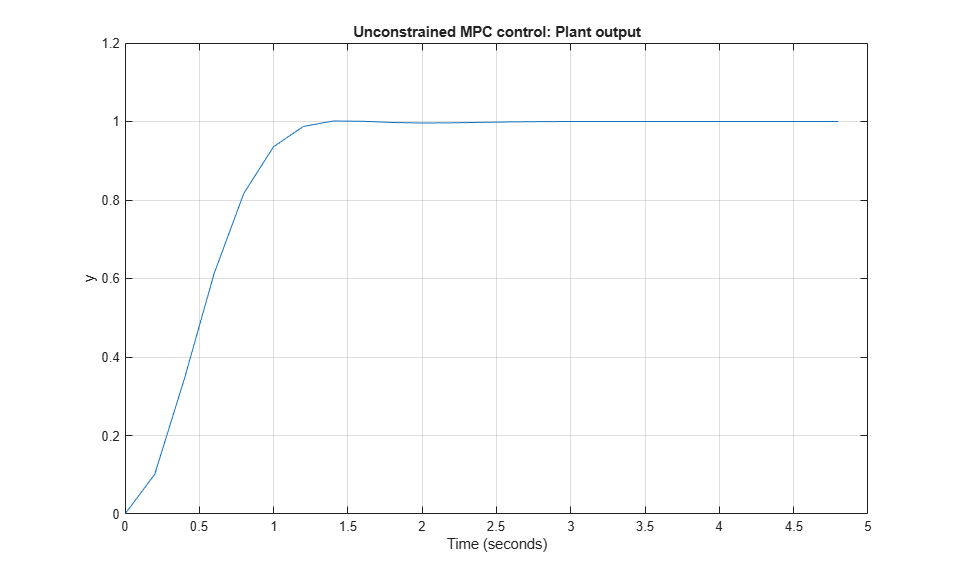Running a closed-loop simulation in which all controller constraints are turned off is easier using `sim`, as you just need to specify 'off' in the `Constraint` field of the related `mpcsimopt` simulation option object.

```SimOptions = mpcsimopt; % create simulation options object SimOptions.Constraints = 'off'; % remove all MPC constraints SimOptions.UnmeasuredDisturbance = d; % unmeasured input disturbance sim(mpcobj,Nf,r,v,SimOptions); % run closed loop simulation ```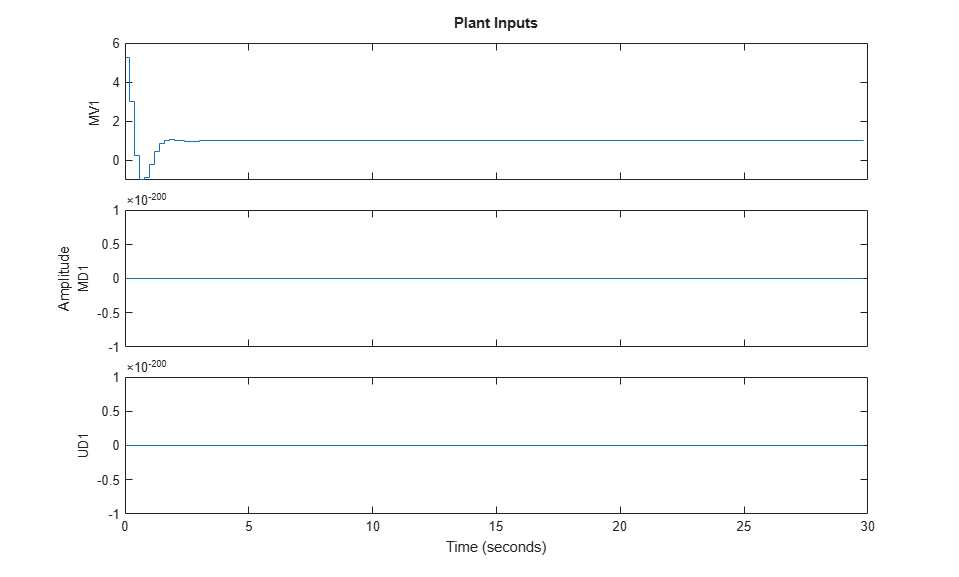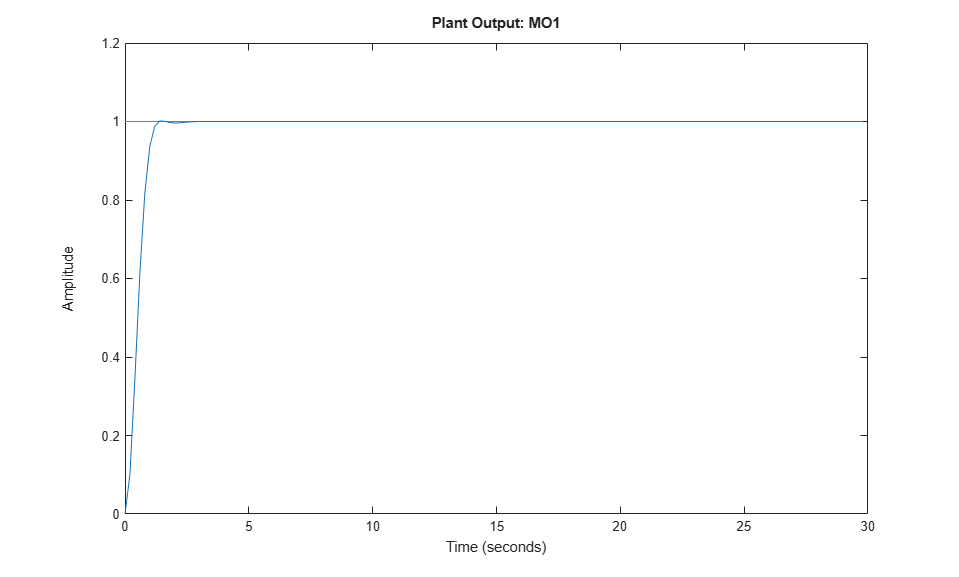Simulink is a graphical block diagram environment for multidomain system simulation. You can connect blocks representing dynamical systems (in this case the plant and the MPC controller) and simulate the closed loop. Besides the fact that the system and its interconnections are represented visually, one key difference is that Simulink can use a wider range of integration algorithms to solve the underlying system differential equations. Simulink can also automatically generate C (or PLC) code and deploy it for real-time applications.

```% To run this part of the example, Simulink(R) is required. Check that % Simulink is installed, otherwise display a message and return if ~mpcchecktoolboxinstalled('simulink') % if Simulink not installed disp('Simulink(R) is required to run this part of the example.') return % end execution here end ```

Recreate the original mpc object with the original constraint and the original default estimator, so you can easily compare results.

```mpcobj = mpc(plantDSS,Ts,10,3); mpcobj.MV = struct('Min',0,'Max',1,'RateMin',-10,'RateMax',10); mpcobj.Model.Disturbance = tf(sqrt(1000),[1 0]); ```
```-->The "Weights.ManipulatedVariables" property of "mpc" object is empty. Assuming default 0.00000. -->The "Weights.ManipulatedVariablesRate" property of "mpc" object is empty. Assuming default 0.10000. -->The "Weights.OutputVariables" property of "mpc" object is empty. Assuming default 1.00000. ```

Extract the state-space matrices of the (continuous-time) plant to be controlled, since they are needed by the Simulink model to be opened.

```[A,B,C,D] = ssdata(plantCSS); % get state-space realization ```

Open the pre-existing Simulink model for the closed-loop simulation. The plant model is implemented with a continuous state space block, and the ode45 integration algorithm is used to calculate its continuous time response.

```%The block inputs are u(t), v(t) and d(t) representing the manipulated % variable, measured input disturbance, and unmeasured input disturbance, % respectively, while y(t) is the measured output. The block parameters % are the matrices forming the state space realization of the continuous-time % plant, and the initial conditions for the 5 states. % The MPC controller is implemented with an MPC controller block, which % has the workspace mpc object |mpcobj| as parameter, the manipulated % variable as output, and then the measured plant output, reference signal, % and measured plant input disturbance as inputs, respectively. % The four Scopes blocks plot the five loop signals, which are also saved % (except the reference signal) by four To-Workspace blocks. open_system('mpc_miso') ```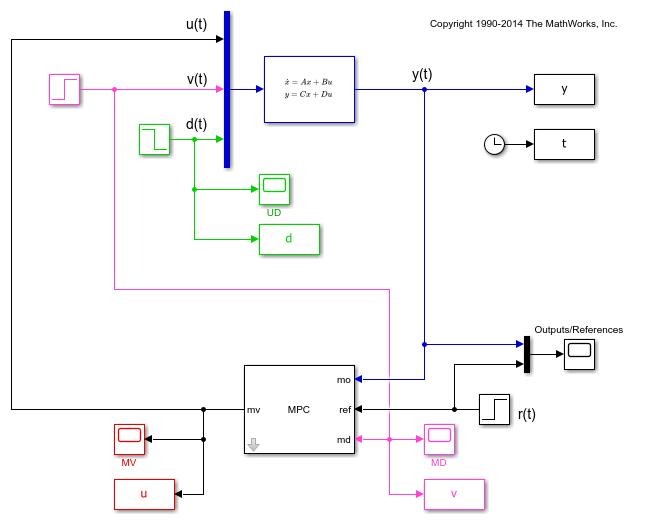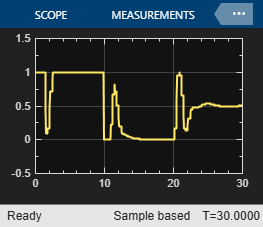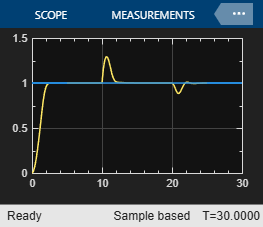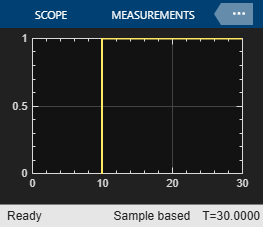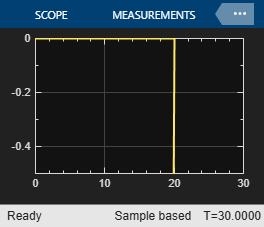Simulate the system using the simulink `sim` command. Note that this command (which simulates a Simulink model, and is equivalent to clicking the "Run" button in the model) is different from the `sim` command provided by the mpc toolbox (which instead simulates an MPC controller in a loop with an LTI plant).

```sim('mpc_miso') ```
``` Assuming no disturbance added to measured output channel #1. -->The "Model.Noise" property of the "mpc" object is empty. Assuming white noise on each measured output channel. ```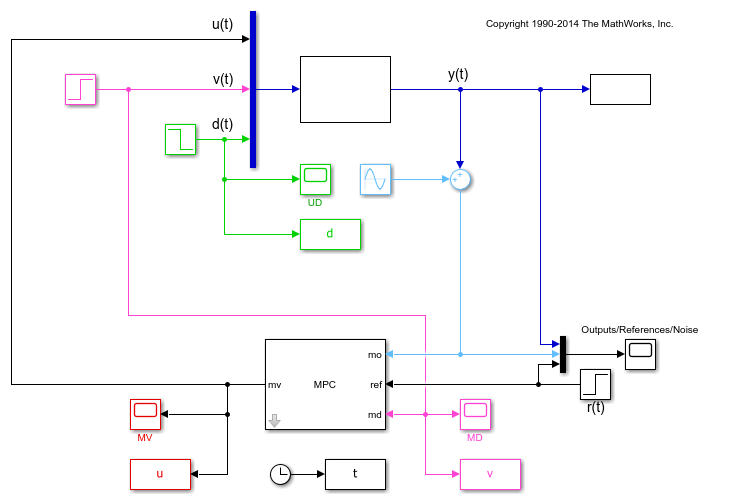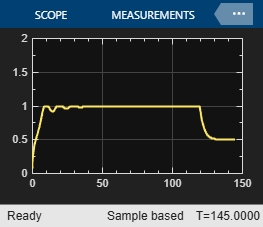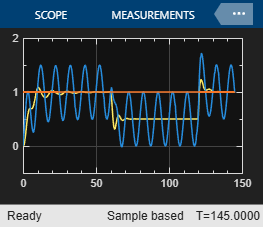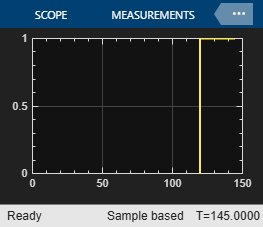The plots in the `Scopes` blocks are equivalent to the ones in figures 1,2 and 3, with minor differences due to the fact that in the first simulation (Figures 1 and 2) the unmeasured disturbance signal was zero, and that is the second simulation (Figure 3) noise was added to the plant input and output. Also note that that, while the MPC `sim` command internally discretizes any continuous plant model using ZOH, Simulink typically uses an integration algorithm (in this example ode45) to simulate the closed loop when a continuous-time block is present.

### Run a simulation with sinusoidal output noise.

Assume output measurements are affected by a sinusoidal disturbance (a single tone sensor noise) of frequency 0.1 Hz on the measured output.

```omega = 2*pi/10; % disturbance radial frequency ```

Open the `mpc_misonoise` Simulink model. It's similar to the 'mpc_miso' model except for the sinusoidal disturbance injected on the measured output. What is also different is that the simulation time is now set to 150 seconds, and that unmeasured and measured input disturbances start to act at after 60 and 120 seconds, respectively. Note that, differently from the previous simulations, the unmeasured disturbance start first. This allows the response to unmeasured disturbance, taking place from 60 to 120 seconds, to be plotted and analyzed more clearly.

```open_system('mpc_misonoise') % open new Simulink model ```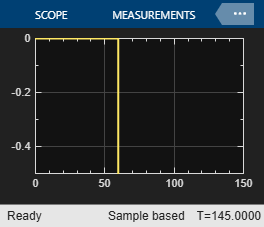Since this noise is expected, specifying it in a measurement noise model will help the state estimator to ignore it, thereby improving the quality of the state estimates and allowing the whole controller to better reject disturbances while tracking the output reference.

```mpcobj.Model.Noise = 0.5*tf(omega^2,[1 0 omega^2]); % measurement noise model ```

Revise the MPC design by specifing a disturbance model on the unmeasured input as a white Gaussian noise with zero mean and variance `0.1`. This allows a better rejection of generic non constant disturbances (at the expense of a worse rejection of input constant disturbances).

```mpcobj.Model.Disturbance = tf(0.1); % static gain ```

Note that when you specify a static gain as a model for the input disturbance, an output disturbance model consisting in a discretized integrator is automatically added to the controller. This helps rejecting constant (and slow varying) output disturbances.

```getoutdist(mpcobj) ```
```-->Assuming output disturbance added to measured output channel #1 is integrated white noise. -->A feedthrough channel in NoiseModel was inserted to prevent problems with estimator design. ans = A = x1 x1 1 B = u1 x1 0.2 C = x1 MO1 1 D = u1 MO1 0 Sample time: 0.2 seconds Discrete-time state-space model. ```

Decrease the weight on the tracking of the output variable, (thereby placing more emphasis on minimizing the rate of change of the manipulated variables).

```mpcobj.weights = struct('MV',0,'MVRate',0.1,'OV',0.005); % new weights ```

By themselves, the new weights would penalize the manipulated variable change too much, resulting in a very low bandwidth (slow) controller. This would result in the output variables lagging behind the reference for many steps. Increasing the predicion horizon to 40 allows the controller to fully take into account the cost of the output error for 40 steps instead of just 10, thereby placing back some emphasis on tracking.

```mpcobj.predictionhorizon = 40; % new prediction horizon ```

Run the simulation for 145 seconds

```sim('mpc_misonoise',145) % the second argument is the simulation duration ```
```-->Assuming output disturbance added to measured output channel #1 is integrated white noise. -->A feedthrough channel in NoiseModel was inserted to prevent problems with estimator design. ```The kalman filter successfully learns to ignore the measurement noise after 50 seconds. The unmeasured and measured disturbances get rejected in a 10 to 20 second timespan. Finally, as expected, the manipulated variable stays in the interval between 0 and 1.

```bdclose('all') % close all open Simulink models without saving any change close all % close all open figures ```